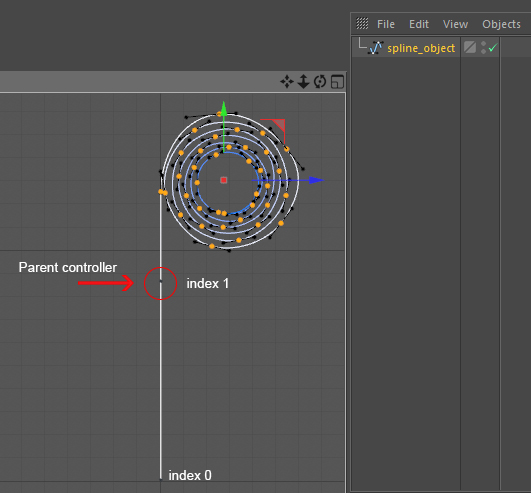# Moving a group of spline points

Hello,

I want to moving group of spline points by just moving one another point as parent controller.
In my example (see screenshot below), I want to control the Y position of all the point after the point 1by just moving the point 1 (using the point 1 like as a parent object)``````def main():
pline_object = op
height = 800
# set the points
spline_object.SetPoint(0, c4d.Vector(0,0, 0))
spline_object.SetPoint(1, c4d.Vector(0,height,0))

obj_pnts = spline_object.GetAllPoints()
for i in xrange(len(obj_pnts)):
if i > 1 :
# What do I make on: obj_pnts[i].y
spline_object.SetPoint(i, c4d.Vector(obj_pnts[i].x, obj_pnts[i].y, obj_pnts[i].z))
``````

Thank you.

I found the solution :

``````def main():
pline_object = op
height = 800

# set the points
spline_object.SetPoint(0, c4d.Vector(0,0, 0))
#spline_object.SetPoint(1, c4d.Vector(0,height,0))

obj_pnts = spline_object.GetAllPoints()
for i in xrange(len(obj_pnts)):
if i > 0 :
interval = height - obj_pnts.y
spline_object.SetPoint(i, c4d.Vector(obj_pnts[i].x,obj_pnts.y+(obj_pnts[i].y-obj_pnts.y)+interval, obj_pnts[i].z))

``````

hello,

happy you found your solution, and thanks for the feedback :)

Cheers
Manuel

MAXON SDK Specialist

MAXON Registered Developer